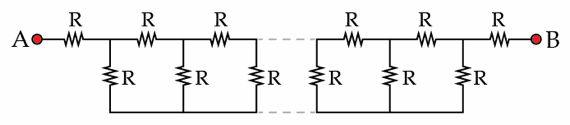# Finite resistance

Consider the following model (see the figure) of a long cable connecting the points A and B. Each resistor in the figure has resistance $R=1~\Omega$. Determine the equivalent resistance $R_{AB}$ in Ohms. Assume the number of resistors to be infinite.×

Problem Loading...

Note Loading...

Set Loading...